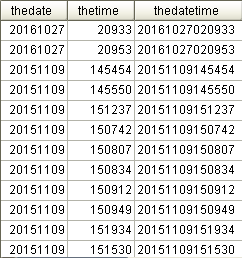### [Informix][SQL]int型別的日期、時間欄位，如何組成yyyyMMddHHmmss格式字串(24小時制、左邊補0)、時間日期格式

• 464
• 0
• 2018-04-27

.``````--theDate,theTime的DataType=int，column_size=4

SELECT
theDate,
theTime,
theDate :: VARCHAR(8) || Lpad(theTime :: VARCHAR(6), 6, '0') AS theDateTime
FROM
TableA;``````

``````SELECT
current as DateTimeNow,
to_char(current,'%Y%m%d') as yyyyMMdd,
to_char(current,'%Y_%m_%d') as yyyy_MM_dd,
to_char(current,'%Y%m%d %H%M%S') as yyyyMMddHHmmss,
to_char(current,'%H%M%S') as HHmmss,
to_char(current,'%H:%M:%S') as HH_mm_ss
FROM
systables
WHERE
tabid= 1; ``````